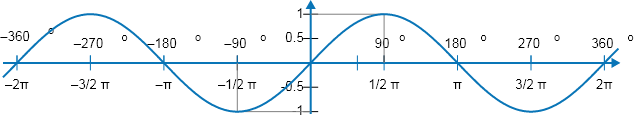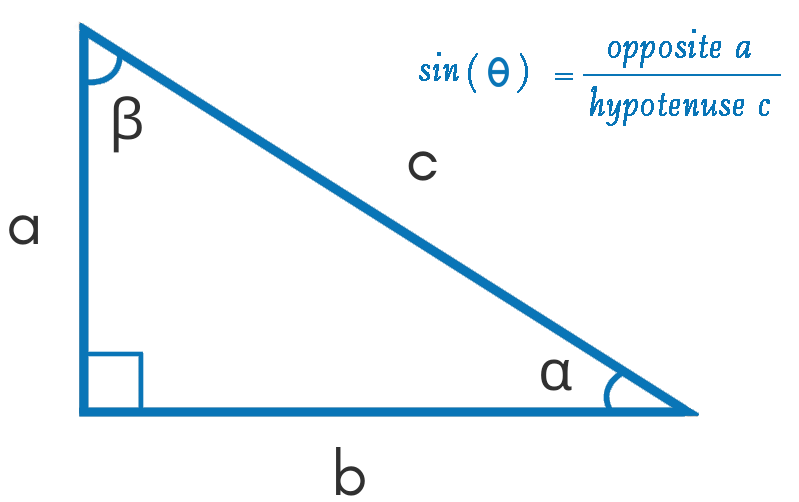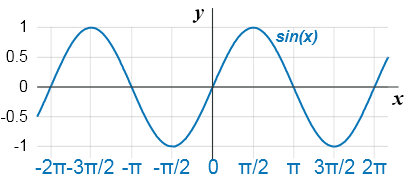Uh Oh! It seems you’re using an Ad blocker!

Since we’ve struggled a lot to makes online calculations for you, we are appealing to you to grant us by disabling the Ad blocker for this domain.# Sine CalculatorAngle (θ)

Table of Content
 1 Sine Formula? 2 Law of sines: 3 How to find the Sine of an Angle? 4 Sin X Graph: 5 Table for Sine: 6 Why Sine is called sine? 7 Are sine functions one to one? 8 Is sine an odd function?
Get The Widget!

Add Sin Calculator to your website to get the ease of using this calculator directly. Feel hassle-free to account this widget as it is 100% free, simple to use, and you can add it on multiple online platforms.

Available on App

The online sine calculator will determine the sine trignometric values for the given angle in degree, radian, or the π radians.

Just take some time out to know how to find sin, how to draw sine graph to represent the sine curve, and much more that will guide you in solving trigonometric functions that involve sine.

## What is Sin in math?

In mathematics, the Sine function is defined in the setting of a right triangle. For a selected angle it is the ratio between the length of the side that is opposite to that angle and the hypotenuse.

### Sine Formula:

• Sine formula is: sin(α) = opposite a / hypotenuse c

However, to solve in sine calculator, there is no need to enter the formula, just simply put the relevant values.

Furthermore, An Online CSC Calculator allows you to find the cosecant (csc) trigonometric function for entered angle it either in degree, radian, or the π radians.

## How to find the Sine of an Angle?

The sine of an angle α in a right-angled triangle can be represented by the ratio between the angle’s opposite side and its hypotenuse. However, you can use the following formula to calculate the sin of an angle and use a sine calculator for quick and easy calculations:

Sine (α) = a / c

Example:

If the length of the opposite side of an angle is 10 and the hypotenuse equal to 2 then the value for sine function can be calculated as follows:

• sin(α) = 10 / 6 = 5 / 2### Sin X Graph:

The sine graph for every possible angle will come in a repeating up/down curve is known as a sine wave. The sine curve will start with an angle of 0, then rises to a value of 1 before decreasing to a value of -1. Then the same pattern will carry on as shown below:## Table for Sine:

Sine values for some common angles are shown in the following table:

 Degrees° Radians sine(x) 0° 0 0 30° π/6 0.50 45° π/4 0.707107 60° π/3 0.866025 90° π/2 1 120° 2π/3 0.866025 135° 3π/4 0.707107 150° 5π/6 0.50 180° π 0

Furthermore, an Online Arcsin Calculator helps you to calculate the arcsin(x) and display the results in radians and degrees.

## How does the Sine Calculator Work?

This sine calculator functioned to follow the sine equation automatically and delivers the sine value in the blink of an eye for any angle. Its working procedure is explained below:

### Input:

• In the first step enter the value of the angel
• Click the “calculate” button.

### Output:

This sine calculator will determine:

• The value of sine either in radians, degrees, or pi (π) radians according to your required angle.
• Make another calculation by clicking on the “re-calculate” option.

## FAQs:

### What are Sine and Cosine for?

The motive of sine and cosine is to solve a triangle to determine the lengths of each of its sides and all its angles. We will apply the sine function when there are two angles and one side. On the other hand, the cosine function will be applied when there are given three sides or two sides with the included angle.

### Why Sine is called Sine?

The word “sine” comes from the Latin language and devised by Robert of Chester of the Arabic jiba, which is the conversion of the Sanskrit word jya-ardha.

### Are Sine Functions One to One?

No Sine function is not one-to-one. when we have to define the inverse functions for sine, the domains are restricted by 0 ≤ x ≤ π.

### Is Sine an Odd Function?

Yes, Sine is an odd function, however, Most functions in trigonometry are neither odd nor even but some functions are one or the other. However, the most convenient way to handle the sine function is the use of a sine calculator.

## Conclusion:

Thankfully, this sine calculator will allow students and professionals to deal with complex angles and dimensions in relatively little time. There’s no need to remember the trigonometric sine equation, just enter the value and let the tool do the calculations accurately.

## References:

From the source of Wikipedia: Right-angled triangle, Traveling and standing waves,  Law of sines.

From the source of Lumen Learning: Graph variations of  sin( x ), Investigating Sinusoidal Functions, Determining Amplitude, Vertical shift of a function.

From the source of Varsity Tutors: Sine Function, Graphing Sine Function, Amplitude and Period of a Since Function.